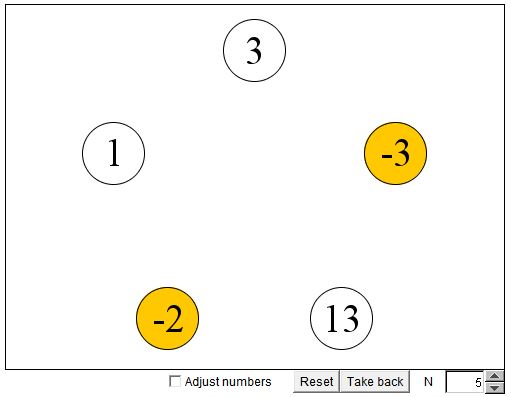# Integer Iterations on Circle III

Here is Problem #3 from the 1986 International Mathematical Olympiad:

To each vertex of a regular pentagon an integer is assigned, so that the sum of all five numbers is positive. If three consecutive vertices are assigned the numbers x, y, z respectively, and y < 0, then the following operation is allowed: x, y, z are replaced by x+y, -y, z+y, respectively. Such an operation is performed repeatedly as long as at least one of the five numbers is negative. Determine whether this procedure necessarily comes to an end after a finite number of steps.

Here is an applet to play with. The applet generally displays five numbers that satisfy the conditions of the problem. You can modify them manually by clicking or dragging (when the box "Adjust numbers" is checked) the mouse next to each number's vertical midline. To perform the algorithmic step click on a negative number.

### If you are reading this, your browser is not set to run Java applets. Try IE11 or Safari and declare the site https://www.cut-the-knot.org as trusted in the Java setup.What if applet does not run?To each vertex of a regular pentagon an integer is assigned, so that the sum of all five numbers is positive. If three consecutive vertices are assigned the numbers x, y, z respectively, and y < 0, then the following operation is allowed: x, y, z are replaced by x+y, -y, z+y, respectively. Such an operation is performed repeatedly as long as at least one of the five numbers is negative. Determine whether this procedure necessarily comes to an end after a finite number of steps.

### If you are reading this, your browser is not set to run Java applets. Try IE11 or Safari and declare the site https://www.cut-the-knot.org as trusted in the Java setup.What if applet does not run?

According to [Engel, p. 6], this was the most difficult problem at the 1986 olympiad. Eleven participants solved the problem, with ten of them coming up with one solution and one following a completely different approach.

On solution was based on the behavior of the function

f(x) = f(x1, x2, x3, x4, x5) = ∑(xk - xk + 2)², x6 = x1, x7 = x2,

and the sum taken from k = 1 through k = 5.

Assume x4 < 0 and consider what happens to f when the algorithm prescribed in the problem has been applied to x4. Denote the new values y1, ..., y5:

 y1 = x1, y2 = x2, y3 = x3 + x4, y4 = -x4, y5 = x5 + x4.

We are going to compute

f(y) - f(x) = ∑(yk - yk + 2)² - ∑(xk - xk + 2

term by term using a² - b² = (a - b)(a + b):

 (y1 - y3)² - (x1 - x3)² = -x4(2x1 - 2x3 - x4), (y2 - y4)² - (x2 - x4)² = 4x4x2, (y3 - y5)² - (x3 - x5)² = 0, (y4 - y1)² - (x4 - x1)² = 4x4x1, (y5 - y2)² - (x5 - x2)² = x4(2x5 + x4 - 2x2).

Summing up all five gives

f(y) - f(x) = 2x4(x1 + x2 + x3 + x4 + x5) < 0,

meaning that f is a strictly decreasing function: f(y) < f(x). Now, f being the sum of squares, is never negative and this is clearly impossible to construct an infinite decreasing sequence of its values, which shows that the iterations must stop, sooner or later.

This solution has been included into the The IMO Compendium. Another solution (by Bernard Chazelle from Princeton, NJ) tells us how soon.

Consider an infinite multiset S of all sums s(k, m), with m > k, where

s(k, m) = xk + ... + xm - 1, k = 1, ..., 5.

An iteration either does not change an element of S, or swaps it with another element; this with the exception of the element to which the iteration applies. The latter element changes its sign. So, from the point of view of set S, each iteration causes just one modification: a change of a sign in a single element. Assuming again that the iteration applies at x4 < 0, the only element of S that undergoes a change is s(4, 5). Since the sum of all x's is positive, there is in S only a finite number of negative elements. Thus the iterations must stop after the number of steps equal to the initial number of negative elements of S.

This solution shows that the numbers need not be integers; it applies to real numbers as well.

The problem and its relation to state-of-th-art mathematical research in celluar automata are the topic of Stanislav Smirnov's chapter in An Invitation to Mathematics. Stanislav was one of the participants of the olympiad who did solve the problem.

### References

1. D. Djukic et al, The IMO Compendium, Springer, 2011 (Second edition)
2. A. Engel, Problem-Solving Strategies, Springer, 1998
3. D. Schleicher, M. Lackmann, An Invitation to Mathematics, Springer, 2011